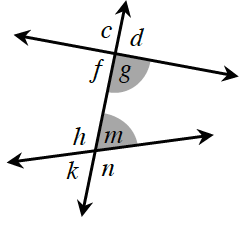### Home > GC > Chapter 3 > Lesson 3.1.3 > Problem3-31

3-31.

Examine the diagram below. Name the geometric relationships of the angles below.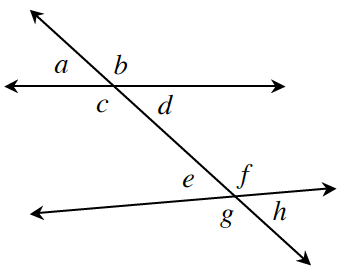1. $d$ and $e$

See More Angle Pair Relationships below.

1. $e$ and $h$

See part (a).

1. $a$ and $e$

Corresponding angles

1. $c$ and $d$

### More Angle Pair Relationships

Vertical angles are the two opposite (that is, non-adjacent) angles formed by two intersecting lines, such as angles $∠c$ and $∠g$ in the diagram at right. $∠c$ by itself is not a vertical angle, nor is $∠g$, although $∠c$ and $∠g$ together are a pair of vertical angles.  Vertical angles always have equal measure.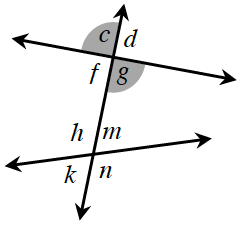Corresponding angles lie in the same position but at different points of intersection of the transversal.  For example, in the diagram at right, $∠m$ and $∠d$ form a pair of corresponding angles, since both of them are to the right of the transversal and above the intersecting line.  Corresponding angles are congruent when the lines intersected by the transversal are parallel.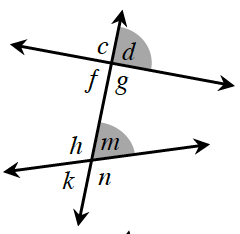$∠f$ and $∠m$ are alternate interior angles because one is to the left of the transversal, one is to the right, and both are between (inside) the pair of lines.  Alternate interior angles are congruent when the lines intersected by the transversal are parallel.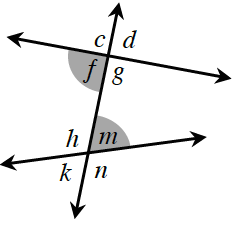$∠g$ and $∠m$ are same-side interior angles because both are on the same side of the transversal and both are between the pair of lines.  Same-side interior angles are supplementary when the lines intersected by the transversal are parallel.